• 若已知运算符之间的优先关系，可按如下步骤构造优先函数： 1、对每个运算符a（包括#在内）令f(a)=g(a)=1 2、如果a⋗b且f(a)<=g(b)令f(a)=g(b)+1 3、如果a⋖b且f(a)>=g(b)令g(b)= f(a)+1 4、如果a≐b而f(a)...
若已知运算符之间的优先关系，可按如下步骤构造优先函数：
1、对每个运算符a（包括#在内）令f(a)=g(a)=1
2、如果a⋗b且f(a)<=g(b)令f(a)=g(b)+1
3、如果a⋖b且f(a)>=g(b)令g(b)= f(a)+1
4、如果a≐b而f(a) ≠g(b)，令min{f(a),g(b)}=max{f(a),g(b)}
5、重复2~4，直到过程收敛。如果重复过程中有一个值大于2n，则表明不存在算符优先函数。

import java.util.Scanner;

/**
* Created by redli on 2017/5/2.
*
* 样例输入1
* --------------------------------------------------
请输入终结符（输入#号结束）：
+*()i#
请输入关系矩阵(#号结束)：
><<><>><><<<<+<>>!>!>>!>!#
-----------------------------------------------------
* 样例输入2
* --------------------------------------------------
请输入终结符（输入#号结束）：
abcd#
请输入关系矩阵(#号结束)：
!!>>!!>!<=<!=!=!#
-----------------------------------------------------
*/
public class Floyd {
public static Scanner in = new Scanner(System.in);
public static StringBuffer terminal = new StringBuffer();
public static StringBuffer matrix = new StringBuffer();
public  static void main(String args[]){
//输入
Input input = new Input();
input.inputTerminal();
input.inputMatrix();

//优先函数
PriorityFunction priority_function = new PriorityFunction();
priority_function.makePriorityFunction();
priority_function.createPriorityFunction();
priority_function.outPriorityFunction();
}

private static class Input{
//输入终结符
public void inputTerminal(){
System.out.println("请输入终结符（输入#号结束）：");
String str = in.next();
while(!str.equals("#")){
if(str.substring(str.length()-1).equals("#")){
terminal.append(str.substring(0,str.length()-1));
str = "#";
} else{
terminal.append(str);
str = in.next();
}
}
}

/*
* 输入关系矩阵
* 输入>，<，=，！（表示为空）
* */
public void inputMatrix(){
System.out.println("请输入关系矩阵(#号结束)：");
String str = in.next();
while(!(str.equals("#"))){
if(!(str.substring(str.length()-1).equals("#"))){
matrix.append(str);
str = in.next();
} else{
matrix.append(str.substring(0,str.length()-1));
str = "#";
}
}
}
}

/*
* 优先函数处理类
* */
private static class PriorityFunction{
int num = terminal.length();
int [][] arr = new int[num];
/*
* 构造优先函数，赋初值1
* */
public void makePriorityFunction(){
for(int i=0; i<2; i++){
for(int j=0;j<num;j++){
arr[i][j] = 1;
}
}
}

/*
*
* 生成优先函数
*
*  */
public void createPriorityFunction(){
int k=1;
int terLength = terminal.length();
while(k!=0){
k = 0;
for(int i=0; i<terminal.length();i++){
for(int j=0; j<terminal.length(); j++){
if(Character.toString(matrix.charAt(i*terLength+j)).equals(">") && arr[i]<=arr[j]){
arr[i]=arr[j]+1;
k=1;
} else if(Character.toString(matrix.charAt(i*terLength+j)).equals("<") && arr[i]>=arr[j]){
arr[j]=arr[i]+1;
k=1;
}
}
}
}
}

/*
* 输出优先函数
* */
public void outPriorityFunction(){
for(int k=0; k<terminal.length(); k++){
System.out.print(terminal.charAt(k)+" ");
}
System.out.println();
for(int i=0; i<2; i++){
for(int j=0; j<terminal.length();j++){
System.out.print(arr[i][j]+" ");
}
System.out.print("\n");
}
}
}
}


展开全文• 使用warshall算法实现的简单优先文法，C++实现的 这是我们课程设计的报告
• 算符优先法中算符优先矩阵的构造需要求出firstTerm以及lastTerm，由于这个是在预测分析法上进行修改的，结构体的定义并未进行修改，其中包含该非终结符a以及其对应的first（即为firstTerm）和follow（即为lastTerm）...
算符优先法中算符优先矩阵的构造需要求出firstTerm以及lastTerm，由于这个是在预测分析法上进行修改的，结构体的定义并未进行修改，其中包含该非终结符a以及其对应的first（即为firstTerm）和follow（即为lastTerm），输入格式如下（以#结束输入）  Z::=E  E::=T|E+T  T::=F|T*F  F::=(E)|i  #
#include<bits/stdc++.h>
using namespace std;
//判断终结符和非终结符 返回1是非终结符 返回0是终结符
int Norterminal(char c)
{
if(c>='A'&&c<='Z')
return 1;
else if(c!=':'&&c!='='&&c!='<'&&c!='>'&&c!=' '&&c!='|')
return 0;

}
struct GRAM
{
//$代表空 string a; string first; string follow; }; struct LIST { int num; string s; }; int main() { GRAM gram; string grammer; cout<<"请输入文法（以#结束）"<<endl; std::vector<string> strN; cin>>grammer;//输入规则 strN.push_back(grammer); char str; const char *ch = "|"; char *result; vector<string> strN1; //存处理过“|”规则的 for(int i=0;i<grammer.length();i++) { str[i]=grammer[i]; } for(int h=grammer.length();h<80;h++) { str[h]=NULL; } result = strtok(str,ch); while(result!=NULL) { strN1.push_back(result); result = strtok(NULL,ch); } for(int i=1;grammer!="#";i++) { cin>>grammer; strN.push_back(grammer); /*处理转换的规则形式输入部分*/ for(int h=0;h<grammer.length();h++) { str[i][h]=grammer[h]; } for(int h=grammer.length();h<80;h++) { str[i][h]=NULL; } result = strtok(str[i],ch); while(result!=NULL) { strN1.push_back(result); result = strtok(NULL,ch); } } /* 获取firstTerm集合 */ //直接获取firstTerm for(unsigned i=0;i<strN.size();i++) { gram[i].a=strN[i]; gram[i].first=""; if(!Norterminal(strN[i])) { gram[i].first=strN[i]; } else { if(!Norterminal(strN[i])) { gram[i].first=strN[i]; } } for(unsigned k=5;k<strN[i].length();k++) { if(strN[i][k]=='|') { if(!Norterminal(strN[i][k+1])) { gram[i].first+=strN[i][k+1]; } else { if(!Norterminal(strN[i][k+2])) { gram[i].first+=strN[i][k+2]; } } } } } int num=strN1.size(); //当第一个字符是非终结符时 for(int i=num-1;i>=0;i--) { for(unsigned l=0;l<strN.size()-1;l++) { if(gram[l].a==strN1[i]) { for(unsigned h=0;h<strN.size()-1;h++) { if(strN1[i]==gram[h].a&&strN1[i]!=strN1[i]) { gram[l].first+=gram[h].first; string ustr(gram[l].first); sort(ustr.begin(), ustr.end()); ustr.erase(unique(ustr.begin(), ustr.end()), ustr.end() ); gram[l].first=ustr; break; } } } } } cout<<"firstTerm集合为："<<endl; for(unsigned i=0;i<strN.size()-1;i++) { for(int k=0;k<gram[i].first.length();k++) { if(gram[i].first[k]==NULL) { gram[i].first.erase(gram[i].first.begin()+k); } } cout<<gram[i].a<<" "<<gram[i].first<<endl; } string term=""; cout<<"终结符为："<<endl; for(unsigned i=0;i<strN.size()-1;i++) { for(int j=0;j<strN[i].length();j++) { if(!Norterminal(strN[i][j])&&strN[i][j]!='$')
term+=strN[i][j];
}
string ustr(term);
sort(ustr.begin(), ustr.end());
ustr.erase(unique(ustr.begin(), ustr.end()), ustr.end() );
term=ustr;
}
cout<<term<<endl;
/*转换规则形式*/
cout<<"转换后的规则形式为："<<endl;
int listnumber =0;
for(unsigned i=0;i<strN1.size()-1&&i-1>=0;i++)
{

if(strN1[i].find("::")==-1)
{

}
cout<<strN1[i]<<endl;
}
/*获取lastTerm*/
//直接获取lastTerm
for(int i=0;i<num-1;i++)
{
for(unsigned l=0;l<strN.size()-1;l++)
{
if(gram[l].a==strN1[i])
{
for(unsigned h=0;h<strN.size()-1;h++)
{
int k=strN1[i].length();
if(!Norterminal(strN1[i][k-1]))
{

gram[l].follow+=strN1[i][k-1];
string ustr(gram[l].follow);
sort(ustr.begin(), ustr.end());
ustr.erase(unique(ustr.begin(), ustr.end()), ustr.end() );
gram[l].follow=ustr;
break;
}
else
{
if(!Norterminal(strN1[i][k-2]))
{
gram[l].follow+=strN1[i][k-2];
string ustr(gram[l].follow);
sort(ustr.begin(), ustr.end());
ustr.erase(unique(ustr.begin(), ustr.end()), ustr.end() );
gram[l].follow=ustr;
break;
}
}
}
}
}
}
for(int i=num-1;i>=0;i--)
{
for(unsigned l=0;l<strN.size()-1;l++)
{
if(gram[l].a==strN1[i])
{
for(unsigned h=0;h<strN.size()-1;h++)
{
int k=strN1[i].length();
if(Norterminal(strN1[i][k-1]))
{

for(unsigned h1=0;h1<strN.size()-1;h1++)
if(gram[h1].a==strN1[i][k-1])
{
gram[l].follow+=gram[h1].follow;
string ustr(gram[l].follow);
sort(ustr.begin(), ustr.end());
ustr.erase(unique(ustr.begin(), ustr.end()), ustr.end() );
gram[l].follow=ustr;
break;
}

}
}
}
}

}
cout<<"lastTerm集合为："<<endl;
for(unsigned i=0;i<strN.size()-1;i++)
{
cout<<gram[i].a<<" "<<gram[i].follow<<endl;
}
int list[term.length()][term.length()];
for(int i=0;i<term.length();i++)
{
for(int j=0;j<term.length();j++)
{
list[i][j]=0;
}
}
cout<<"相等的关系为："<<endl;
for(int i=num-1;i>=0;i--)
{
for(int k=0;k<strN1[i].length()&&k+1<strN1[i].length();k++)
{
if(Norterminal(strN1[i][k]))
{
if(!Norterminal(strN1[i][k-1])&&!Norterminal(strN1[i][k+1]))
{
cout<<strN1[i][k-1]<<strN1[i][k+1]<<endl;;
for(int h=0;h<term.length();h++)
{
if(strN1[i][k-1]==term[h])
for(int g=0;g<term.length();g++)
{
if(strN1[i][k+1]==term[g])
{
list[h][g]=1;
}
}
}
}
}
else
{
if(!Norterminal(strN1[i][k+1]))
{
cout<<strN1[i][k]<<strN1[i][k+1]<<endl;
for(int h=0;h<term.length();h++)
{
if(strN1[i][k]==term[h])
for(int g=0;g<term.length();g++)
{
if(strN1[i][k+1]==term[g])
{
list[h][g]=1;
}
}
}
}
}
}
}
//cout<<"小于关系为："<<endl;
for(int i=0;i<num-1;i++)
{
for(int k=0;k<strN1[i].length();k++)
{
if(!Norterminal(strN1[i][k]))
{
if(Norterminal(strN1[i][k+1]))
{
for(int h=0;h<term.length();h++)
{
if(strN1[i][k]==term[h])
{
for(int j=0;j<num-1;j++)
{
if(strN1[i][k+1]==gram[j].a)
{
for(int g=0;g<term.length();g++)
{
for(int f=0;f<gram[j].first.length();f++)
if(gram[j].first[f]==term[g])
{
list[h][g]=2;
}
}
}
}
}
}

}
}
}

}
//大于关系
for(int i=0;i<num-1;i++)
{
for(int k=0;k<strN1[i].length();k++)
{
if(Norterminal(strN1[i][k]))
{
if(!Norterminal(strN1[i][k+1]))
{
for(int h=0;h<term.length();h++)
{
if(strN1[i][k+1]==term[h])
for(int j=0;j<num-1;j++)
{
if(strN1[i][k]==gram[j].a)
{
for(int g=0;g<term.length();g++)
{
for(int f=0;f<gram[j].first.length();f++)
if(gram[j].follow[f]==term[g])
{
list[g][h]=3;
}
}
}
}
}

}
}
}
}
for(int i=0;i<term.length();i++)
{
for(int j=0;j<term.length();j++)
{
cout<<list[i][j]<<" ";
}
cout<<endl;
}

cout<<"算符优先矩阵为："<<endl;
cout<<"    |";
for(int i=0;i<term.length();i++)
{
cout<<setw(10)<<term[i];
cout<<"|";
}
cout<<endl;
cout<<"____________________________________________________________________________"<<endl;

for(int i=0;i<term.length();i++)
{
printf("%2c",term[i]);
cout<<"  "<<"|";
for(int j=0;j<term.length();j++)
{
if(list[i][j]==0)
cout<<setw(10)<<" "<<"|";
if(list[i][j]==1)
cout<<setw(10)<<"="<<"|";
if(list[i][j]==2)
cout<<setw(10)<<"<"<<"|";
if(list[i][j]==3)
cout<<setw(10)<<">"<<"|";

}
cout<<endl;
cout<<"____________________________________________________________________________"<<endl;

}

} 
运行结果如下：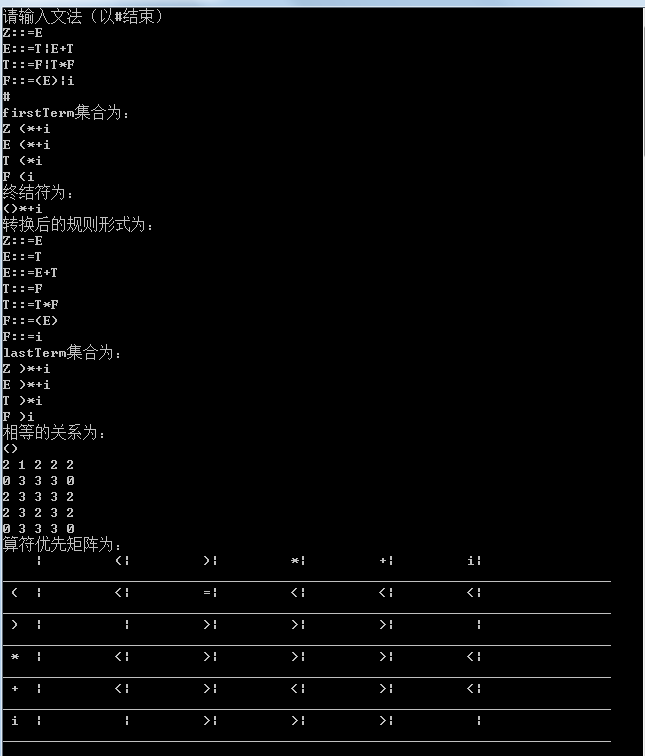展开全文• 2.2.2 构造文法的简单优先关系矩阵 7 2.3 分析器的构造 7 第三章 逻辑设计 9 3.1系统的组织与基本工作流程 9 3.2 总体结构逻辑结构图 9 第思章 软件功能设计 11 4.1 软件功能分析 11 4.1.1 判定文法是否为简单优先...
• 输入算符优先文法，输出FIRSTVT、LASTVT、算符优先关系表 对输入串，输出分析过程
• 编译原理：算符优先分析文法
编译原理：算符优先分析法
语法分析有自上而下（推导）和自下而上（归约）分析法两种，而算符优先分析法是自上而下分析法中的一种，应用也比较普遍，但是值得注意的是，其不是规范归约（最左归约）。
一般情况，算符优先分析法的流程如下： 求解FIRSTVT集和LASTVT集→构造优先关系矩阵→进行句型的“归约”操作。
下文所用文法如下： E→E+T |T T→T*F | F F→(E) | i
FIRSTVT集和LASTVT集
这里我们需要与FIRST集和FOLLOW集合做一下区分： （1）FIRST集和FOLLOW集： ①用于LL(1)文法的预测分析表构造 ②FIRST集和FOLLOW集中均为终结符号集合，但是FOLLOW集中没有ε （2）FIRSTVT集和LASTVT集： ①用于算符优先文法中的优先关系矩阵的构造 ②FIRSTVT集和LASTVT集均为终结符号集合
FIRSTVT集
*定义+求法：通过定义我们可以得出以下几点： ① U→b…，b进入FIRSTVT(U) ②U→V…，FIRSTVT(V)进入FIRSTVT(U) ③U→Vb…，b进入FIRSTVT(U)
（注意：U和V：非终结符号；b：终结符号）
例解 FIRSTVT(E)={+,* , ( , i } FIRSTVT(T)={ * , ( , i } FIRSTVT(F)={ ( , i }
LASTVT集
定义+求法：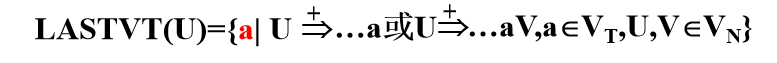通过定义我们可以得出以下几点： ①U->…a，a进入LASTVT（U） ②U->…V，LASTVT（V）进入LASTVT（U） ③U->…aV，a进入LASTVT（U）
（注意：U和V：非终结符号；a：终结符号）
例解 LASTVT(E)={+ , * , ) , i } LASTVT(T)={ * , ) , i } LASTVT(F)={ ) , i }
优先关系矩阵
如果要构造优先关系矩阵，我们需要明确两个相继出现的终结符号a和b之间的优先关系(a与b之间可有一个非终结符)，一旦确定了这种优先关系，就可以用它来确定“可归约串”进行归约。
①a·>b（a的优先级高于b的优先级）：当且仅当G中含有产生式：U→…Ub…，且任意的a∈LASTVT（U），a·>b成立。 ②a<·b（a的优先级低于b的优先级）：当且仅当G中含有产生式：U→…aU…，且任意的b∈FIRSTVT（U），a<·b成立。 ③a=·b（a的优先级等于b的优先级）：当且仅当G中含有产生式：U→…ab…或U→aVb…
NOTE： ①优先级高的先参加归约！ ②优先关系不是对偶的，也就是说：a<·b≠b·>a
例解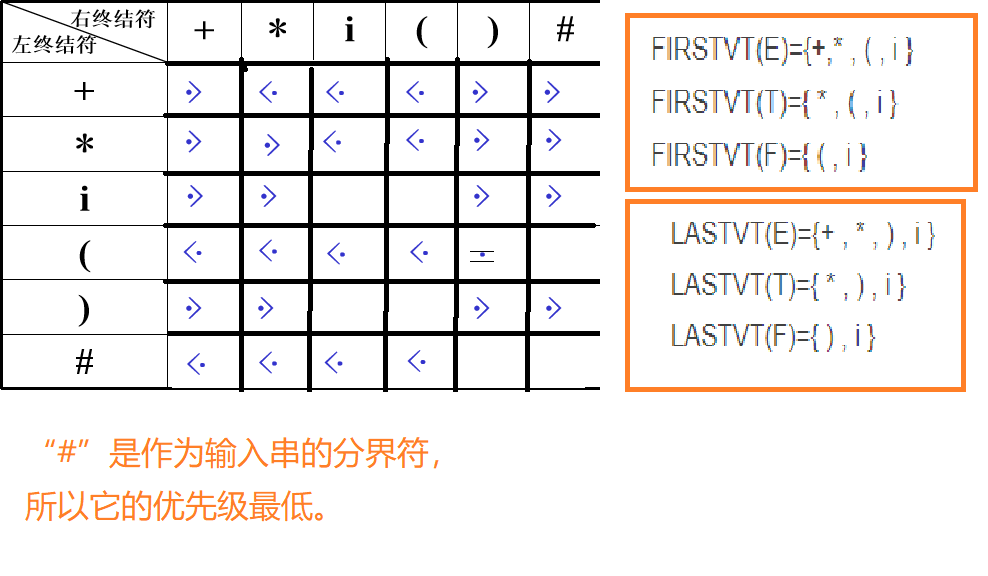归约
在算符优先分析法中采用的是归约最左素短语的方式进行归约。
预备知识
概念问题
（1）短语：一棵子树的所有叶子自左至右排列起来形成一个相对于子树根的短语。 （2）直接短语：仅有父子两代的一棵子树，其所有叶子自左至右排列起来所形成的符号串。 （3）句柄：一个句型的分析树中最左那棵只有父子两代的子树的所有叶子的自左至右排列。 （3）素短语：至少包含有一个终结符号，并且除它之外，不再包含其它素短语。 （4）最左素短语：任意句型最左边的素短语为该句型的最左素短语。 举例：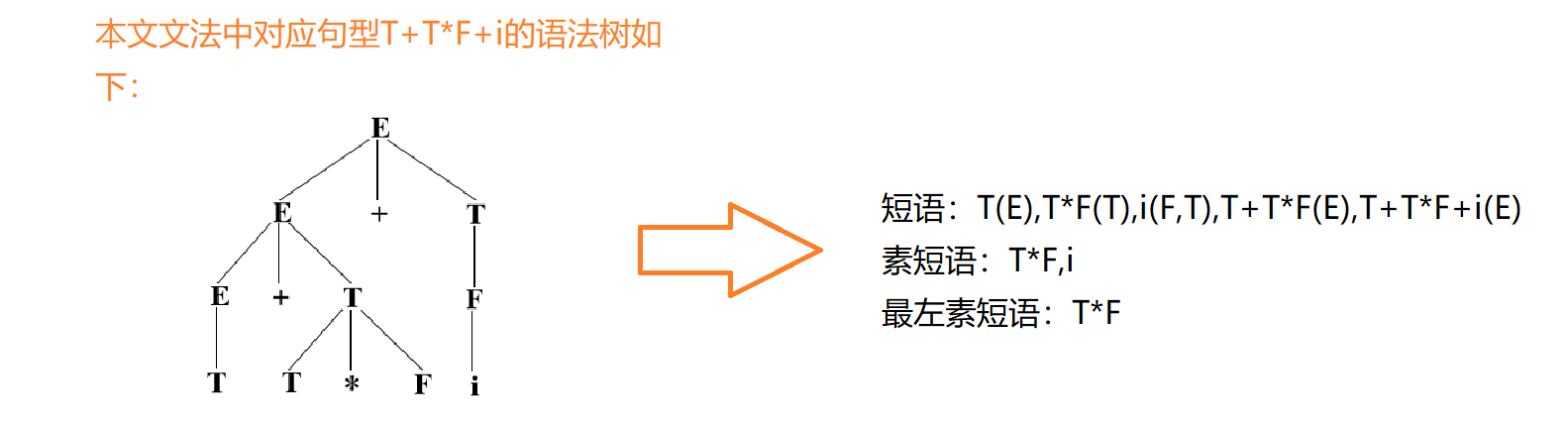最左素短语的特征 （1）算符文法句型的一般形式：#N1a1N2a2……NnanNn+1# 其中，Ni∈VN, ai∈VT； （2）定理：一个算符优先文法的句型的最左素短语是满足下列条件的最左子串：NjajNj+1aj+1……NiaiNi+1
其优先关系为：aj=.aj+1 ，aj+1=. aj+2, …, ai-1 =. ai；且：aj-1<.aj， ai >. ai+1
将子串左右邻接符号一起考虑：aj-1NjajNj+1aj+1……NiaiNi+1ai+1 它们的优先关系表示为：aj-1<.aj=. aj+1=. ……=.ai>.ai+1
则<.和>.之间用等号连接的部分（aj=. aj+1=. ……=.ai）即为最左素短语，可以进行归约。
注意： Nj和Ni+1属于最左素短语，而aj-1和ai+1 不属于最左素短语。
例解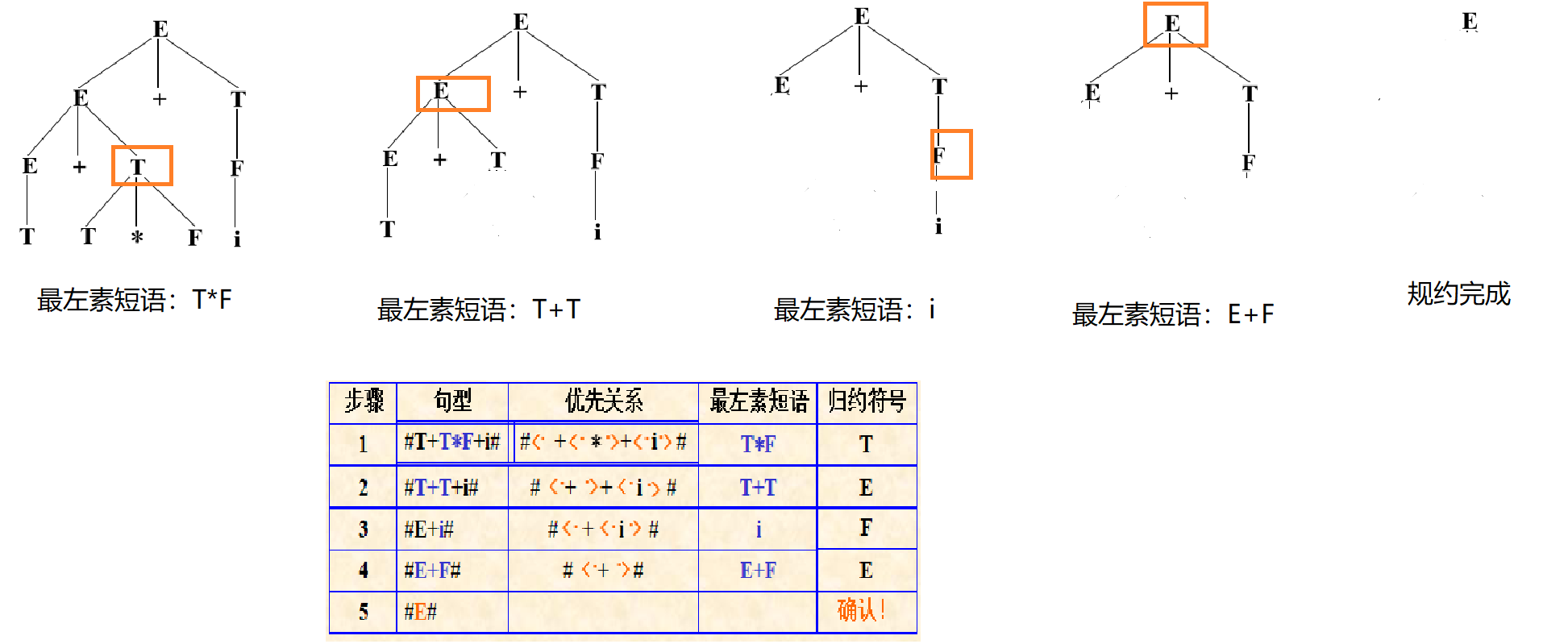至此，算符优先分析已经完成。
展开全文• Firstvt 找Firstvt的三条规则：如果要找A的Firstvt，A的候选式中出现： A->a…，即以终结符开头，该终结符入Firstvt A->B…，即以非终结符开头，该非终结符...前面是终结符，则终结符入Firstvt 算符优先关系表 实例 2.
Firstvt
找Firstvt的三条规则：如果要找A的Firstvt，A的候选式中出现： A->a…，即以终结符开头，该终结符入Firstvt A->B…，即以非终结符开头，该非终结符的Firstvt入A的Firstvt A->Ba…，即先以非终结符开头，紧跟终结符，则终结符入Firstvt
Lastvt
找Lastvt的三条规则：如果要找A的Lastvt，A的候选式中出现： A->…a，即以终结符结尾，该终结符入Lastvt A->…B，即以非终结符结尾，该非终结符的Lastvt入A的Lastvt A->…aB，即先以非终结符结尾，前面是终结符，则终结符入Firstvt
算符优先关系表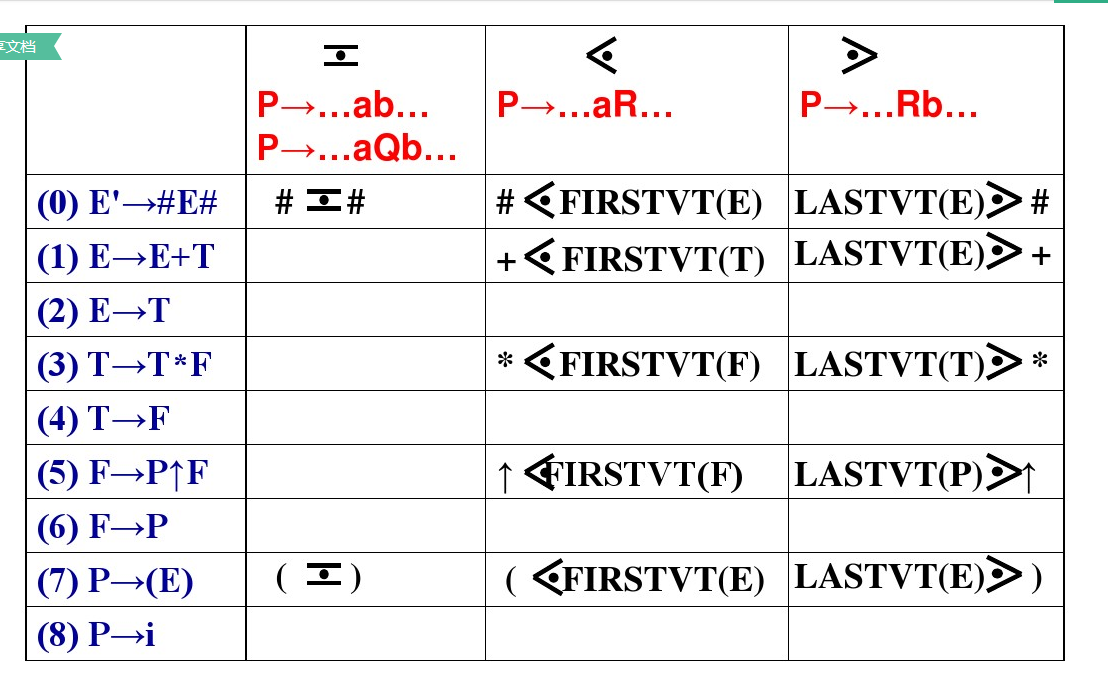实例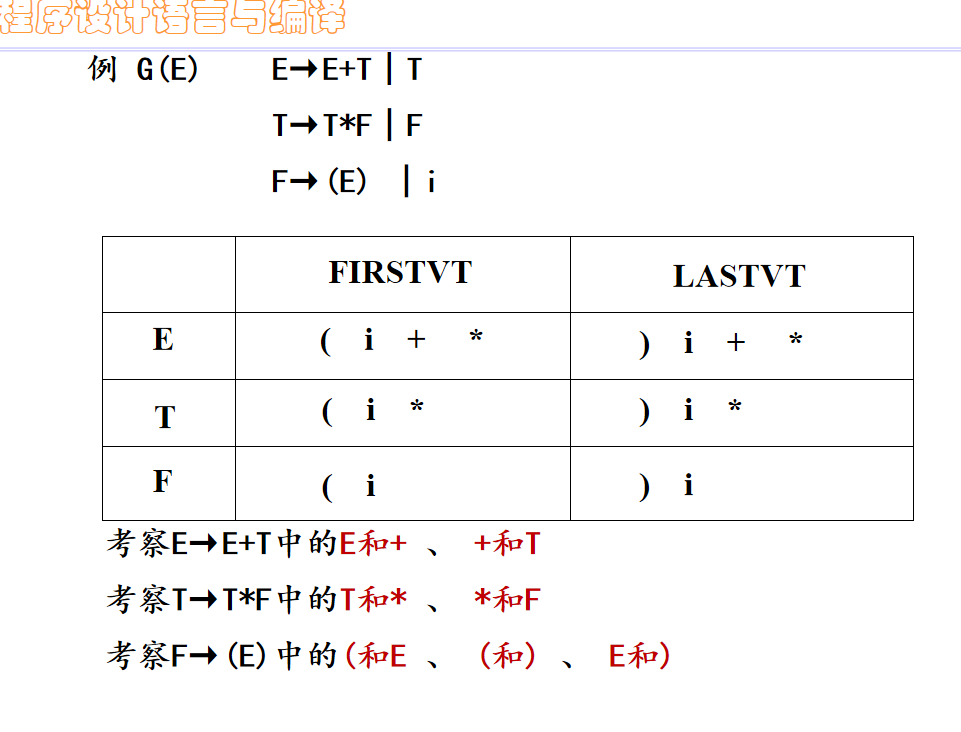2.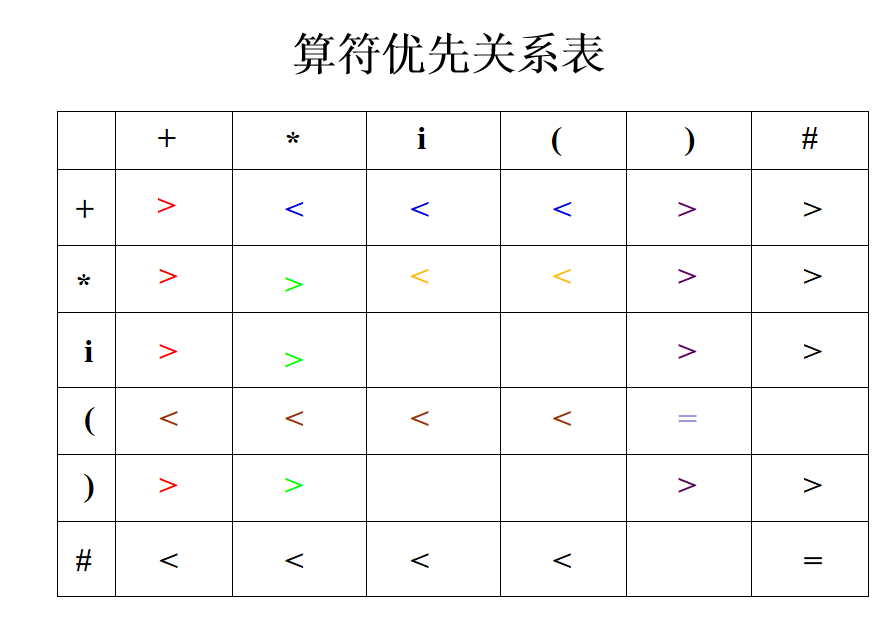展开全文• 在算符优先分析法中，文法终结符之间的优先关系是用优先矩阵表示的，这样需要占用大量的内存空间，当文法有n个终结符时，就需要（n+1）^2个内存单元，因此，在实际实现中使用优先函数来代替优先矩阵表示优先关系。...
• 在邻接矩阵中，可以如下表示顶点和边连接关系： 说明： 将顶点对应为下标，根据横纵坐标将矩阵中的某一位置值设为1，表示两个顶点向联接。 图示表示的是无向图的邻接矩阵，从中我们可以发现它们的分布关于斜对角线...
• 2）采用由定义构造算符优先关系集合并构造矩阵 由以上公式，先得到对应的FIRSTVT与LASTVT集合，再依次遍历文法找到所需的…aV…或者…Vb…产生式，与FIRSTVT（V）或者LASTVT（V）卡氏积，得到>,<,=的算符优先...java
• 最易于实现的一种移动归约分析方法，叫做算符优先分析法，而更一般的移动归约分析方法叫做LR分析法，LR分析法可以用作许多自动的语法分析器的生成器。 短语：文法G[S]，αβδ是文法G的一个句型，S=>*αAδ且A=>+β...
• 算符优先分析法 1.基本概念 算符文法(OG)：文法G中没有形如A=&gt;···BC···的产生式，其中B、C为非终结符，则G为算符文法（operator grammar）。 也就是说产生式的右部不能出现两个非终结符相邻，就...
• 最易于实现的一种移动归约分析方法，叫做算符优先分析法， 而更一般的移动归约分析方法叫做LR分析法，LR分析法可以用作许多自动的语法分析器的生成器。 短语：文法G[S]，αβδ是文法G的一个句型，S=>*αAδ且A=>+β...编译原理
• NULL 博文链接：https://128kj.iteye.com/blog/1663164
• 算符优先分析不是一种规范...对于任何两个可能相继出现的终结符 a 和 b ，它们之间的优先关系无非 >、=、<三种关系。 需要注意的是a<b，并不意味着 b > a，a=b并不意味着 b = a 。这是因为与它们在规...
• ## 【编译原理】算符优先算法

万次阅读 多人点赞 2018-05-10 20:48:55
G[E]:E→E+T∣T T→T*F∣FF→(E)∣i构造该算符优先文法的优先关系矩阵或优先函数；输入串应是词法分析的输出二元式序列，即某算术表达式“实验项目一”的输出结果。输出为输入串是否为该文法定义的算术表达式的判断...
• 简单优先分析法 1.基本概念 通过语法树来理解这三个概念更加简单： 文法G1[S]： S→AB A→bB A→Aa B→a B→Sb 语法树 短语：若S=*=&gt;αAδ且A=+=&gt;β，则称β是相对于非终结符A的句型αβδ...
• 输入任意文法，输出优先矩阵初始状态，通过矩阵转置，相乘等的输出，构造简单优先矩阵
• https://github.com/Ggmatch/The-principle-to-compile体验算法优先分析法能达到的效果算符优先分析法只考虑算符（广义为终结符）之间的优先关系，例如若有文法G为： （1）E->E+E （2）E->E*E （3）E->编译原理
• 这种分析方法首先要规定运算符之间(确切地说是终结符之间)的优先关系和结合性质,然后借助这种关系,比较相邻运算符的优先级来确定句型的可归约串并进行归约。 下面,以表达式的文法为例,说明采用这种分析法分析符号串 ...
• 简单优先分析算法  编译原理简单优先分析算法是一种自下而上的分析算法，本例是编译原理第三版何炎祥中一个简单优先分析算法的例子，未给出分析过程分析代码，读者可以参考我的语法分析器中输出代码，写出此算法的...编译原理 c++
• 文法的简单优先关系矩阵： 是编写一个简单优先关系算法，用于识别文法的句子，或句型； 输入 输入句子或句型 输出 判断句子或句型是否符合文法G[S]： 如果符合文法输出...
• 什么是行优先和列优先？ 故名思意，当我们表示一个矩阵的时候把行放在前面就是行优先，把列放在前面就是列优先。 ... ，用一个列优先的平移矩阵表示为，两个矩阵关系也不难看出其实是互为转...
• typedef struct ArcCell{//表征图的连接关系 VRType adj; //连接关系 ArcCell *info; //附加信息 }ArcCell,AdjMatrix[MAX_VERTEX_NUM][MAX_VERTEX_NUM]; typedef struct{ VertexType vexs[MAX_VERTEX_NUM];//...数据结构
• 首先看一下什么是算符文法 算符文法 设有文法G，若G中没有形如U —> …VW…的规则，其中VW为非终结符，则称G为...定义任何两个终结符号之间的优先关系 设G是一个算符文法，a和b是任意两个非终结符，P、Q、R是非...
• 刚做完的。简单优先文法的判定以及分析器的构造。代码可以用，但觉得不够完美，像是没有句子分析的过程。编译课程设计
• 算符优先分析程序和测试用例 算术表达式的文法： E E+T | E-T | T T T*F | T/F | F F（E）| i
• 提出了一种语言判断矩阵的元素与优先权重的含参数的逻辑关系，并对参数的选取进行了分析，最后给出了算例说明．...